Function Repository Resource:

# ScorerGiZero

Find zeros of the ScorerGi function

Contributed by: Jan Mangaldan
 ResourceFunction["ScorerGiZero"][k] represents the kth zero of the Scorer function Gi(x). ResourceFunction["ScorerGiZero"][k,x0] represents the kth zero less than x0.

## Details

Mathematical function, suitable for both symbolic and numerical manipulation.
N[ResourceFunction["ScorerGiZero"][k]] gives a numerical approximation so long as the specified zero exists.
ResourceFunction["ScorerGiZero"][k] represents the kth zero less than 0.
ResourceFunction["ScorerGiZero"] can be evaluated to arbitrary numerical precision.

## Examples

### Basic Examples (1)

Evaluate numerically:

 In:=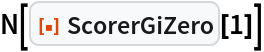Out=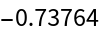### Scope (3)

Evaluate to high precision:

 In:=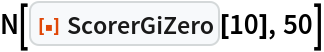Out=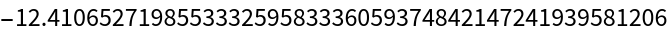Find the second zero of Gi(x) less than -500:

 In:=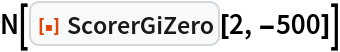Out=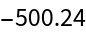In:=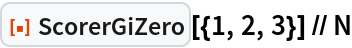Out=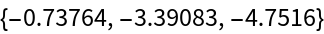### Applications (1)

Display zeros of the ScorerGi function over a subset of the reals:

 In:=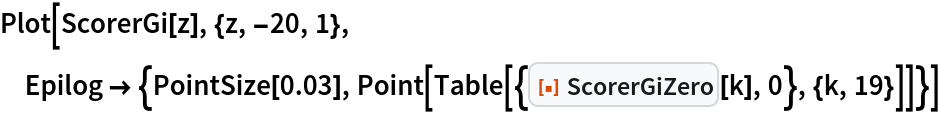Out=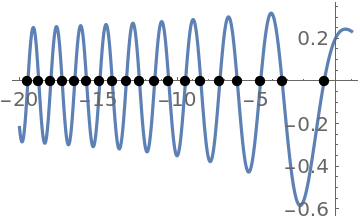## Version History

• 1.0.0 – 07 January 2021